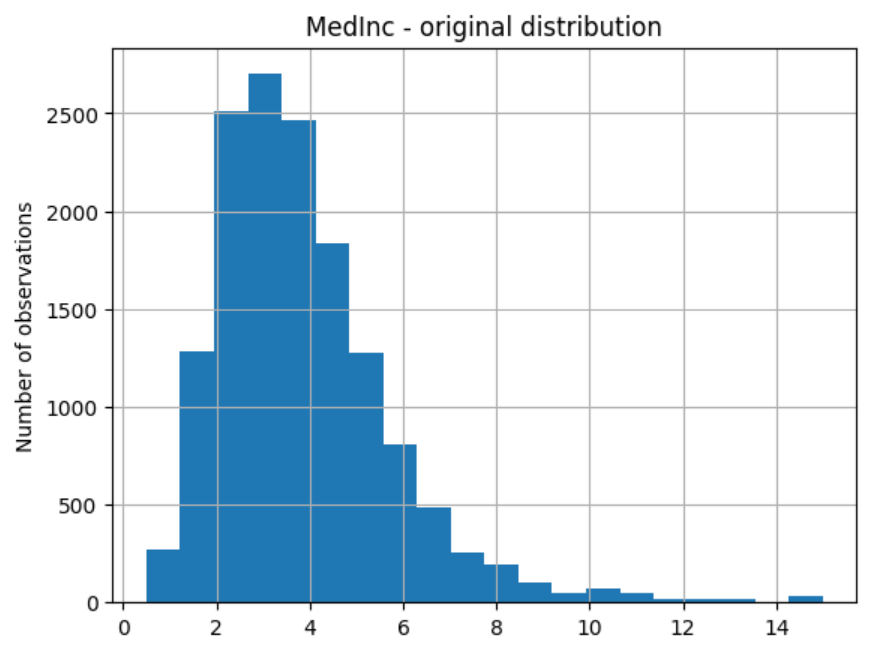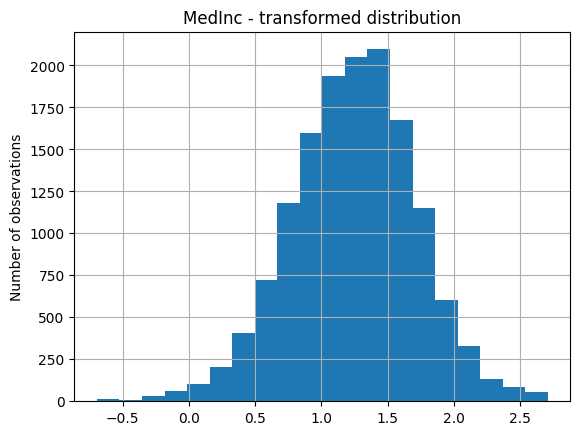# LogCpTransformer#

The `LogCpTransformer()` applies the transformation log(x + C), where C is a positive constant.

You can enter the positive quantity to add to the variable. Alternatively, the transformer will find the necessary quantity to make all values of the variable positive.

## Example#

Let’s load the California housing dataset that comes with Scikit-learn and separate it into train and test sets.

```import pandas as pd
import matplotlib.pyplot as plt
from sklearn.model_selection import train_test_split
from sklearn.datasets import fetch_california_housing

from feature_engine import transformation as vt

X, y = fetch_california_housing( return_X_y=True, as_frame=True)

# Separate into train and test sets
X_train, X_test, y_train, y_test =  train_test_split(
X, y, test_size=0.3, random_state=0)
```

Now we want to apply the logarithm to 2 of the variables in the dataset using the `LogCpTransformer()`. We want the transformer to detect automatically the quantity “C” that needs to be added to the variable:

```# set up the variable transformer
tf = vt.LogCpTransformer(variables = ["MedInc", "HouseAge"], C="auto")

# fit the transformer
tf.fit(X_train)
```

With `fit()` the `LogCpTransformer()` learns the quantity “C” and stores it as an attribute. We can visualise the learned parameters as follows:

```# learned constant C
tf.C_
```
```{'MedInc': 1.4999, 'HouseAge': 2.0}
```

Applying the log of a variable plus a constant in this dataset does not make much sense because all variables are positive, that is why the constant values C for the former variables are possible.

We will carry on with the demo anyways.

We can now go ahead and transform the variables:

```# transform the data
train_t= tf.transform(X_train)
test_t= tf.transform(X_test)
```

Then we can plot the original variable distribution:

```# un-transformed variable
X_train["MedInc"].hist(bins=20)
plt.title("MedInc - original distribution")
plt.ylabel("Number of observations")
```And the distribution of the transformed variable:

```# transformed variable
train_t["MedInc"].hist(bins=20)
plt.title("MedInc - transformed distribution")
plt.ylabel("Number of observations")
```## More details#

You can find more details about the `LogCpTransformer()` here:

All notebooks can be found in a dedicated repository.